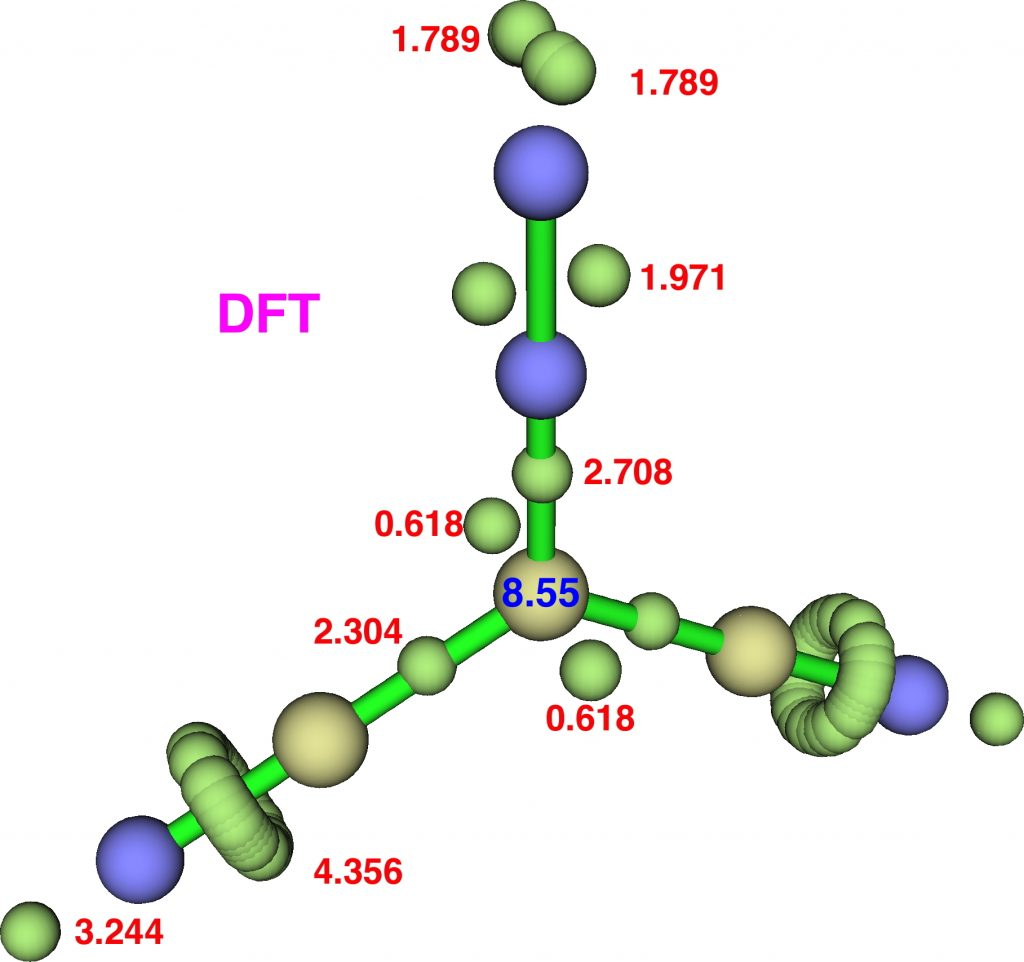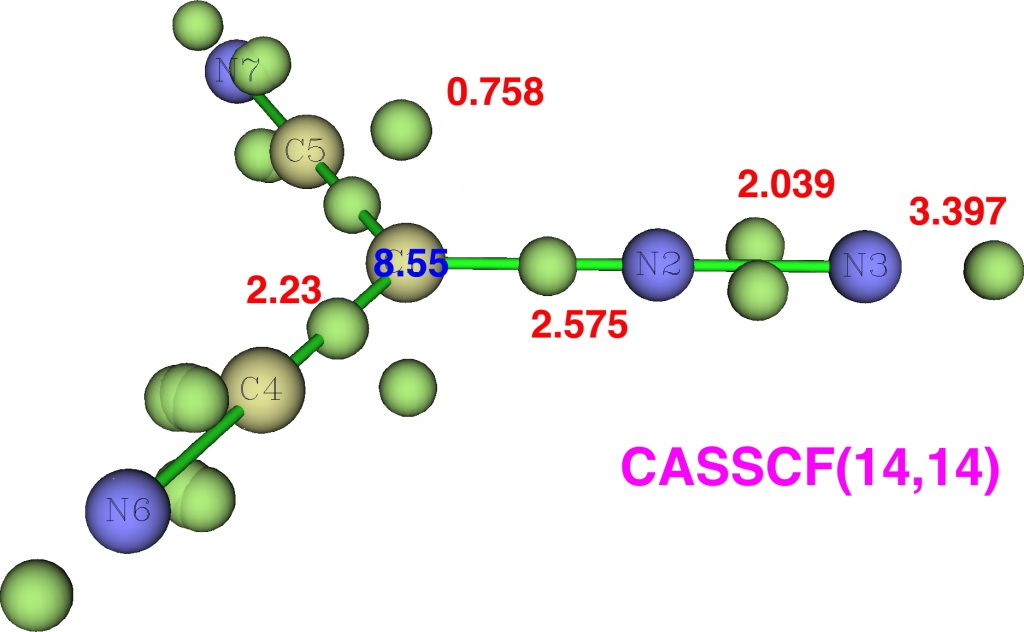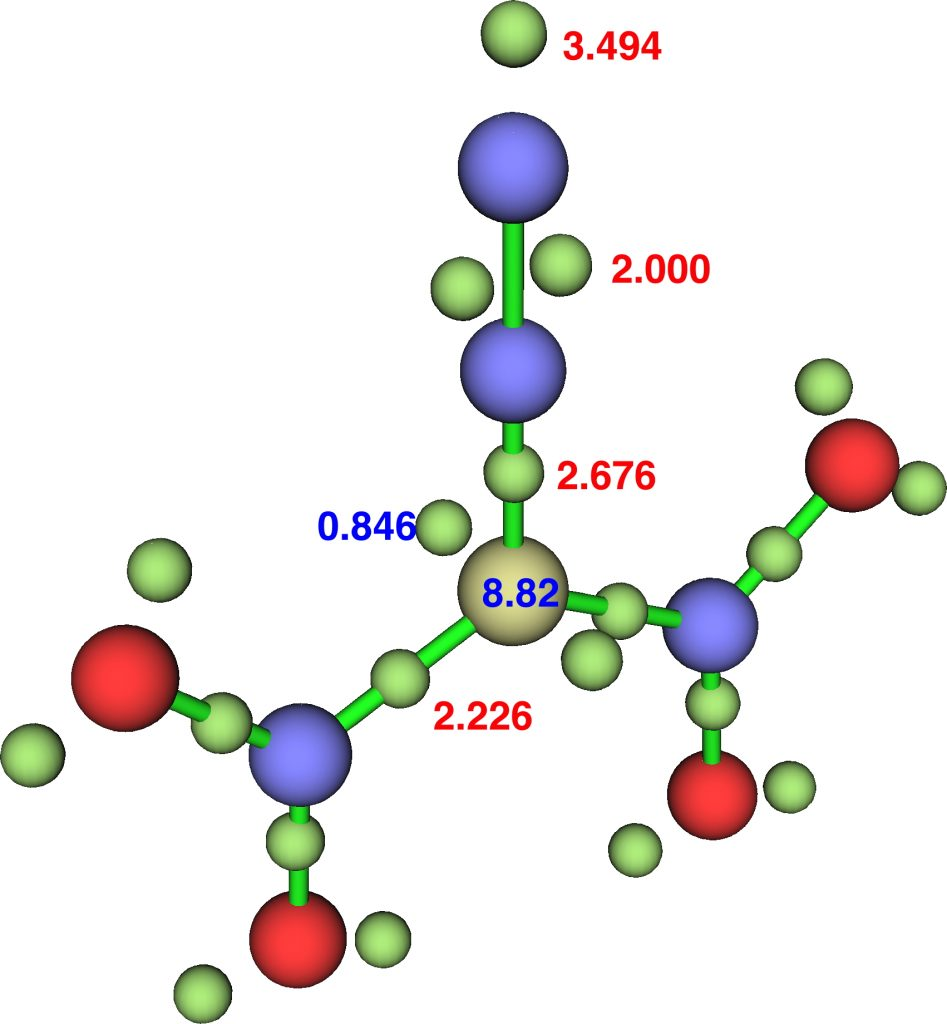## Can any hypervalence in diazomethanes be amplified?

In the previous post, I referred to a recently published review on hypervalency which introduced a very simple way (the valence electron equivalent γ) of quantifying the effect. Diazomethane was cited as one example of a small molecule exhibiting hypervalency (on nitrogen) by this measure. Here I explore the effect of substituting diazomethane with cyano and nitro groups.Firstly, dicyanodiazomethane. NBO analysis reveals the following atom bond indices; C, 3.810; N 3.834; N 2.971. Compare these values to diazomethane itself, C, 3.716; N 3.802; N 2.907 and you can see that the carbon bond index has increased slightly. The ELF basin integrations (below) which also take into account the “lone pair” on carbon are: C, 8.55, N, 6.65, N, 7.52 (DFT), again compared with diazomethane as C, 8.16; N, 6.59; N, 7.52. The CASSCF(14,14) result is very similar.So the “γ(C)” has increased from 8.2 to 8.55. Next, dinitrodiazomethane;The NBO bond indices are C, 3.8203; N 3.8255; N 2.9802 and ELF integrations C, 8.82, N, 6.68, N, 7.49 (DFT).So “γ(C)”  increases along the series 8.16 → 8.55 → 8.82, whereas “γ(N)” changes as 6.59 → 6.65 → 6.68, a smaller effect. Whilst 8.82 is still some way off the value of γ(N)=10 quoted for diazomethane, dinitrodiazomethane is still a pretty good candidate for hypervalent carbon. The question now is whether even larger values of “γ(C)” can be identified in other molecules.

FAIR data for all calculations is available at DOI: 10.14469/hpc/3476. The quotes in “γ(C)” indicate it is calculated here using ELF integrations rather than charge maps.

## References

1. M.C. Durrant, "A quantitative definition of hypervalency", Chemical Science, vol. 6, pp. 6614-6623, 2015. http://dx.doi.org/10.1039/C5SC02076J